# The first programAt the spa
Photo: Holly Vickery

### Working out the tip in a restaurant

Say we want a program that will figure out the tip for a meal, in an American restaurant. It's usually 15%.

Adela, how do you compute a tip?Well, I'd take the meal cost, whatever it was. What is it?

Uh, let's say \$25.OK. The tip is 25 × 0.15. That's 3.75.

Then I'd take the tip, 3.75, and add it to the meal cost, of 25.

That would give a total, 28.75

Great!

• Multiply meal cost by 15%.
• Then add to meal cost to get the total to pay.

That's a program, a set of instructions Adela used to do the task.

To do the task, Adela had to get some data: the meal cost. Then she could do her calculations. The last step of Adela's program was to tell us what the result was.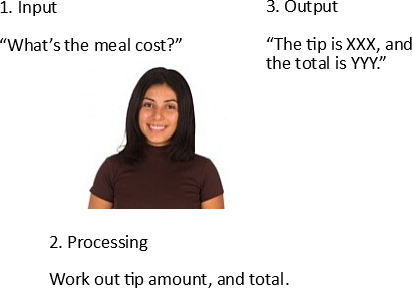Adela's smart, so the program could be a bit sloppy, and she could work out what to do. Here's the program again:

• Multiply meal cost by 15%.
• Then add to meal cost to get the total to pay.

The program doesn't tell Adela to ask us for the meal cost. She figured out that she needed that, though, and asked. The program didn't say she should tell us the results, but she worked out that she should.

Writing the program for Adela to run is pretty easy.

### Computers are stupid

We can't use Adela's program with a computer, because computers are really, really, really stupid. They need every step spelled out. Miss one, or get it wrong, and the program won't work.Truth Goat: You won't believe how stupid computers are.

Here's a program that a computer might run. (It's pseudocode, not in a real programming language.)

• Ask a human for a number. Put that in [meal cost].
• Multiply [meal cost] by 0.15. Put that in [tip amount].
• Add [meal cost] and [tip amount]. Put that in [total].
• Tell the human [tip amount] and [total].

It's much longer than Adela's program:

• Multiply meal cost by 15%.
• Then add to meal cost to get the total to pay.

Why is it longer? Because you need to tell the computer all the deets, or the program won't work.

That's what programmers do. Tell a stupid stupid stupid machine all the deets to work something out.

You can run the program with this button. Try it, with a number, like 50.

Now try it again, but this time type the word "fifty" instead of the number 50. Will the computer be smart enough to know what you wanted?

Nope. Stupid computer.

### Variables

Computers are so stupid, they forget what they did, from one moment to the next. Here are the first two lines of the program again.

• Ask a human for a number. Put that in [meal cost].
• Multiply [meal cost] by 0.15. Put that in [tip amount].

By the time the computer starts running the second line, it's forgotten about the first.

`[meal cost]` and `[tip amount]` are variables. A variable a tiny chunk of computer memory, with a name.

`[meal cost]` links the two lines together. The first line puts something into `[meal cost]`. The second gets something out of `[meal cost]`.

### Ray wonders how stupid computers are

Here's the program again.

• Ask a human for a number. Put that in [meal cost].
• Multiply [meal cost] by 0.15. Put that in [tip amount].
• Add [meal cost] and [tip amount]. Put that in [total].
• Tell the human [tip amount] and [total].Ray

OK, so what if we took out part of the first line, the piece that put the human's data into a variable.

• Ask a human for a number. (Something deleted.)
• Multiply [meal cost] by 0.15. Put that in [tip amount].

Would the program work?

Well, it would run. It wouldn't get the right answer, since `[meal cost]` would stay at zero.Ethan

But, it would still ask for a number?

Yes, because the program says to:

• Ask a human for a number.
• Multiply [meal cost] by 0.15. Put that in [tip amount].Ethan

What would it do with the number it got from a human?

Throw it away, because the code doesn't tell it to do anything with the number.Ray

Wow, that's super-duper stupid.

Yes.

Many bugs are from programmers forgetting how stupid computers are. They miss steps that are so obvious to humans that we don't even think about them. But they must be spelled out to computers.Would the computer know something was wrong with the program?

Ooo, good question! No, it wouldn't. It would run happily, giving wrong answers every time.

So far...

• A program is a set of steps that a computer runs.
• Programmers use variables to link the steps together.
• Computer are stupid.

One more thing. The 0.15 in the line:

• Multiply [meal cost] by 0.15. Put that in [tip amount].

That's called a constant, since it's a value that never changes.

### To Excel

Let's Excelize that. We want a worksheet that starts like this: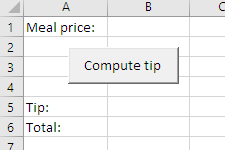The user types a number into cell B1, then clicks the "Compute tip" button.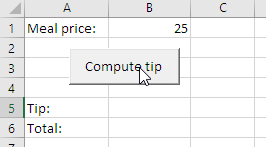The computer works out the answers, and outputs them: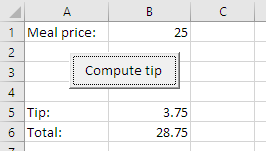Let's make that.

Start Excel, and open a blank workbook. Put the labels in cells A1, A5, and A6.

You turned on the developer tools, right? You saw how to do that earlier.

Now we'll:

• Make a place for some code to live.
• Make a button that runs the code.

#### Make a sub

A sub, or subroutine, is a chunk of code with a name. Like this:

• Sub DemonGoat()
•
• 'Code goes here.
•
• End Sub

You can link a sub to a button, so what when the button is clicked, the sub runs.

There's a special tool you'll use to type in code. It's called the Visual Basic Editor (VBE). One way to get to VBE is with View Code in the Developer tab: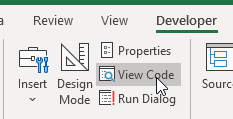I prefer to use the keyboard shortcut. On Windows it's Alt+F11. There's a similar shortcut on the Mac.

VBE opens, and looks something like this: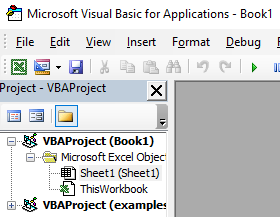The window on the left is called the Project Explorer. Best to keep that open. If it's not there, or you accidentally close it, you can open it with the View menu.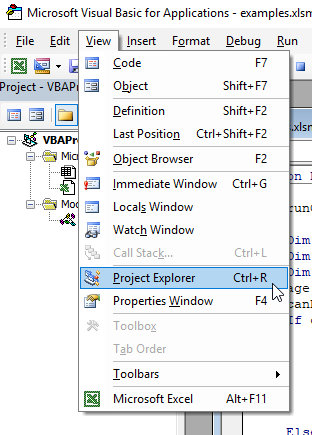Notice the shortcut, Ctrl+R. Shortcuts are good.

The Project Explorer shows where you can put your subs. There are three common places: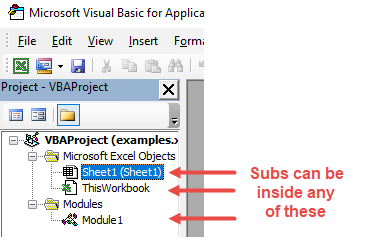Let's put all of our subs inside the sheet.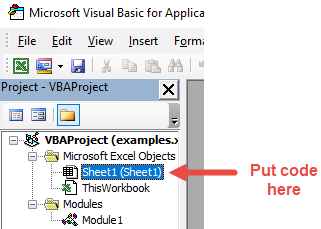Double-click on Sheet1, and you'll see an editor for subs for Sheet1.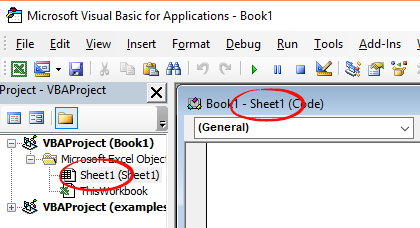Here's what the editor will look like when there's some code in it.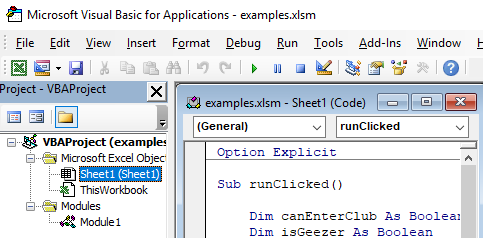Ray

What happens if I accidentally put code in one of the other places?

No problem. Cut-and-paste the code into the Sheet1 place. Make sure you get the whole thing, from the `Sub` to the `End Sub`.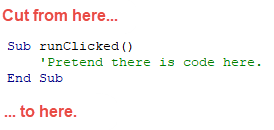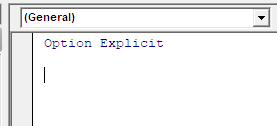The line `Option Explicit` should be there, because you set the Require Variable Declaration option earlier: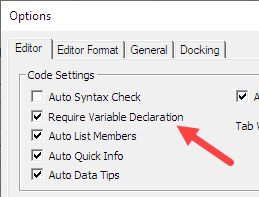If you forgot to set the option, do it now with Tools | Options (select Options from the Tools menu). If you still don't see `Option Explicit`, type it in.

Important! `Option Explicit` should be the first line in every program. It helps avoid bugs. We'll talk about why later.

Put this code after `Option Explicit`:

• Sub runClicked()
•   Dim sMealPrice As Single
•   Dim sTipAmount As Single
•   Dim sTotal As Single
•   ' Input meal price.
•   sMealPrice = Cells(1, 2)
•   ' Compute tip and total.
•   sTipAmount = sMealPrice * 0.15
•   sTotal = sMealPrice + sTipAmount
•   ' Output.
•   Cells(5, 2) = sTipAmount
•   Cells(6, 2) = sTotal
• End Sub

We'll go through it in a bit.

Notice the code lives inside a `Sub`, named `runClicked`. All of our code will live in `Sub`s.

OK, now your code has a home. Onward!

pause,

#### Making a button to run the code

Go to the Developer tab. Click Insert, and choose Button from the form controls.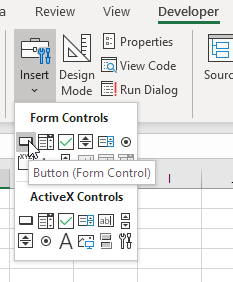Then click in the worksheet where you want the button to appear. You can move the button later, if you want.

You'll get a dialog window like this: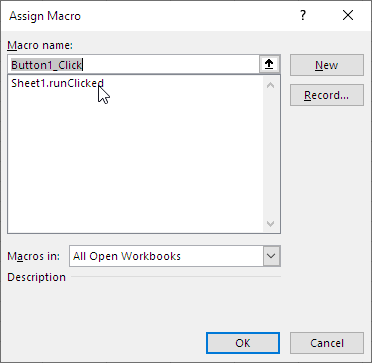You want `runClicked` to run when the user clicks the button. Click on `runClicked`, and then click OK: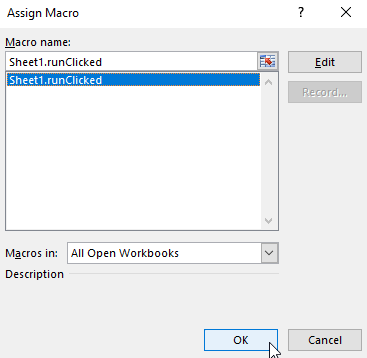I notice it says `Sheet1.runClicked`.

Right! Good noticing.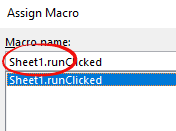That's because an Excel workbook can have more than one worksheet. We'll usually have just one, called `Sheet1`.

After you click OK, you'll see the button on your worksheet. Something like this: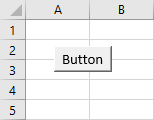Now you've linked the button to your code. So when someone clicks on the button, `runClicked` will run.

Give it a try. Put in a meal amount, and click the button.

#### The button caption

We want the word Run on the button, so let's fix that.

Go into design mode: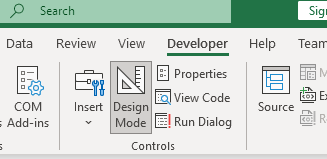Right-click on the button, and select Edit text: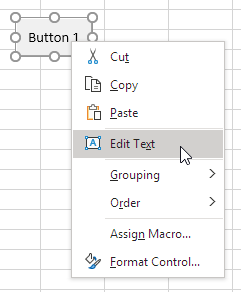This will let you set the button's caption. Change it to Run.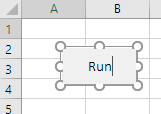The small circles show that you are editing the button. When you're done, click off the button, somewhere in the worksheet.

To move a button, enter design mode, right-click on the buttin, and then press Escape to hide the menu. Move the mouse around until you see a move arrow.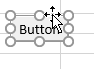Now drag the button where you want it.

#### Excel workbooks with VBA in them need to be saved in a special way. When you save, choose Excel Macro-Enabled Workbook: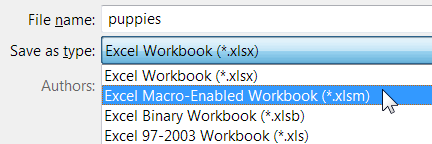Notice the extension: `xlsm`.

If you save a workbook as a regular Excel file (extension: `xlsx`), you could lose your code. Argh! Don't do that.

When you open an XLSM file, you'll get warnings: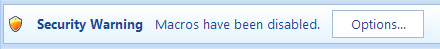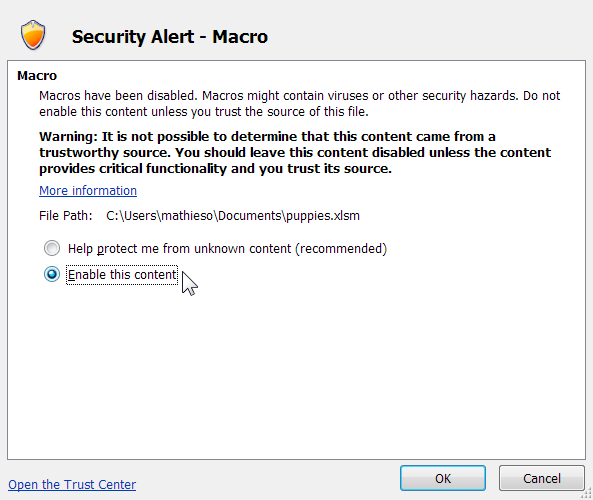You get to decide whether you trust the file. If it's code you wrote, it should be safe. Unless you're trying to hack yourself.

### How the code works

• Dim sMealPrice As Single
• Dim sTipAmount As Single
• Dim sTotal As Single
• ' Input meal price.
• sMealPrice = Cells(1, 2)
• ' Compute tip and total.
• sTipAmount = sMealPrice * 0.15
• sTotal = sMealPrice + sTipAmount
• ' Output.
• Cells(5, 2) = sTipAmount
• Cells(6, 2) = sTotal

The first three lines tell the computer that we need three variables, what the names of the variables are, and that we want to put numbers in them (that's what `Single` means; more later). This is called declaring the variables.Georgina

I'm guessing that the `s` in the variable names, like `sTotal`, has to do with them being `Single`?

Right. It's called Hungarian naming. More later.

After these lines run, computer memory looks like this: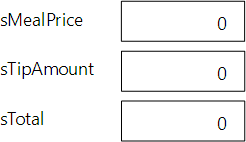Variables in memory

When you declare a variable, VBA automatically sets the variable's value to 0. Not all languages do this. In those languages, you have to set the value to 0 yourself.

### Input

Let's check out the next two lines.

• Dim sMealPrice As Single
• Dim sTipAmount As Single
• Dim sTotal As Single
• ' Input meal price.
• sMealPrice = Cells(1, 2)
• ' Compute tip and total.
• sTipAmount = sMealPrice * 0.15
• sTotal = sMealPrice + sTipAmount
• ' Output.
• Cells(5, 2) = sTipAmount
• Cells(6, 2) = sTotal

The first one is a comment. It explains to a human what the code below it does. Comments begin with a single quote ('). The computer ignores everything after the quote.

The next line says "Take what is in the cell at row 1, column 2, and put it into the variable `sMealPrice`. If the cell at row 1, column 2 had 25 in it: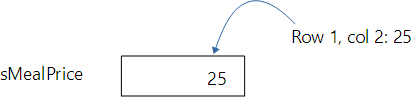Copy a value from a cell in a worksheet, into a variable in memory

Here's that line again:

•     sMealPrice = Cells(1, 2)

Important! The = does not mean what it means in math. It's something else entirely in programming. It says: "Take the value of the thing on the right, and copy it into the thing on the left."So the = is a bit confusing, then.

Yes, I agree. It would better if it looked like an arrow:

•     sMealPrice <- Cells(1, 2)

That's clearer: take the value of the thing on the right, and copy it into the thing on the left.

That's not the way VBA is, though. You have to use =.

This is called an assignment statement, since it assigns a value to a variable. So an assignment statement always has the form:

•     to_here = from_here

### Computation

Here's the code again:

• Dim sMealPrice As Single
• Dim sTipAmount As Single
• Dim sTotal As Single
• ' Input meal price.
• sMealPrice = Cells(1, 2)
• Up to here
• ' Compute tip and total.
• sTipAmount = sMealPrice * 0.15
• sTotal = sMealPrice + sTipAmount
• ' Output.
• Cells(5, 2) = sTipAmount
• Cells(6, 2) = sTotal

We talked about declaring variables, and getting the user's input into a variable.

Let's look at the next three lines:

• Dim sMealPrice As Single
• Dim sTipAmount As Single
• Dim sTotal As Single
• ' Input meal price.
• sMealPrice = Cells(1, 2)
• ' Compute tip and total.
• sTipAmount = sMealPrice * 0.15
• sTotal = sMealPrice + sTipAmount
• ' Output.
• Cells(5, 2) = sTipAmount
• Cells(6, 2) = sTotal

A comment, then more assignment statements. Take the thing on the right, and copy it to the thing on the left.

The thing on the right is an expression. It's a computation of some kind.So the * means multiply?

Yes, it does.A question. The code is:

• sTipAmount = sMealPrice * 0.15
• sTotal = sMealPrice + sTipAmount

Do we need the variable `sTipAmount`? Could we do this instead?

• sTotal = sMealPrice + sMealPrice * 0.15

Yes! Great thinking, Adela! We could do that.

We wouldn't have `sTipAmount`, though, which we need for the output.

### Output

Let's look at the last bit of the code:

• Dim sMealPrice As Single
• Dim sTipAmount As Single
• Dim sTotal As Single
• ' Input meal price.
• sMealPrice = Cells(1, 2)
• ' Compute tip and total.
• sTipAmount = sMealPrice * 0.15
• sTotal = sMealPrice + sTipAmount
• ' Output.
• Cells(5, 2) = sTipAmount
• Cells(6, 2) = sTotal

What's this code do?Ray

Two assignment statements. Take what is on the right, and put it into what is on the left. The things on the left are cells on the worksheet.

So this code copies the variables `sTipAmount` and `sTotal` into the worksheet, so the user can see them.

Right! Those lines output the variables' values.

### Once more with feeling

OK, let's go through the code again. Explain what the pieces of code do, in your own words. Then compare it with what the virtual students think.

Here's some code:

•     Dim sMealPrice As Single
•     Dim sTipAmount As Single
•     Dim sTotal As Single
Reflect

What does the code do?

If you were logged in as a student, the lesson would pause here, and you'd be asked to type in a response. If you want to try that out, ask for an account on this site.Ray

The computer makes three variables in its memory, with the names given. `sMealPrice`, `sTipAmount`, and `sTotal`.

Good. Next:

•     sMealPrice = Cells(1, 2)
Reflect

What does this code do?

If you were logged in as a student, the lesson would pause here, and you'd be asked to type in a response. If you want to try that out, ask for an account on this site.Georgina

Take the value in the cell at row 1, column 2. Put it in the variable `sMealPrice`.

That's an assignment statement.

It's how the program gets input, data from the user.

Nice. Next:

•     sTipAmount = sMealPrice * 0.15
•     sTotal = sMealPrice + sTipAmount
Reflect

What does this code do?

If you were logged in as a student, the lesson would pause here, and you'd be asked to type in a response. If you want to try that out, ask for an account on this site.Ethan

Take whatever is in the variable `sMealPrice`, and multiply it by 0.15. Put the result in `sTipAmount`.

Then take what is in `sMealPrice`, add `sTipAmount`, and put that in `sTotal`.

Excellent. Last bit:

•     Cells(5, 2) = sTipAmount
•     Cells(6, 2) = sTotal
Reflect

What does this code do?

If you were logged in as a student, the lesson would pause here, and you'd be asked to type in a response. If you want to try that out, ask for an account on this site.Take `sTipAmount`, and put its value into the worksheet cell at row 5, column 2. Then put `sTotal` into row 6, column 2.